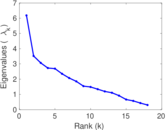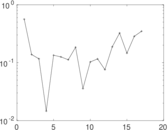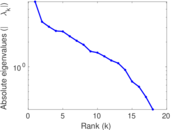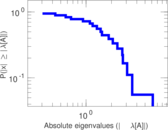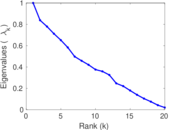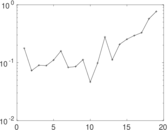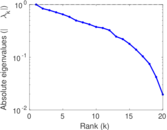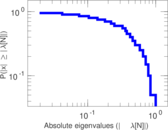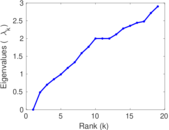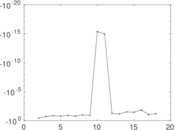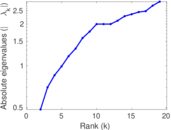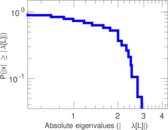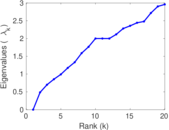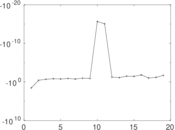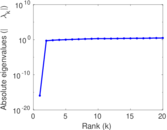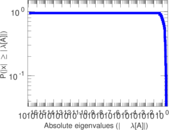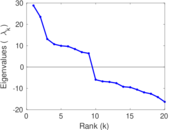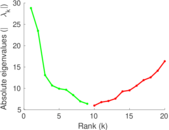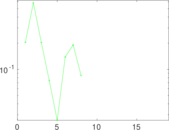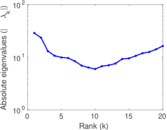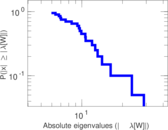This bipartite network contains person–company leadership information between companies and 20 corporate directors. The data was collected in 1962. Left nodes represent persons and right nodes represent companies. An edge between a person and a company shows that the person had a leadership position in that company.

 Code `BC` Internal name `brunson_corporate-leadership` Name Corporate leaderships Data source https://github.com/corybrunson/triadic AvailabilityDataset is available for download Consistency checkDataset passed all tests Category Affiliation network Node meaning Person, company Edge meaning Leadership Network formatBipartite, undirected Edge typeUnweighted, no multiple edges

## Statistics

 Size n = 44 Left size n1 = 20 Right size n2 = 24 Volume m = 99 Wedge count s = 453 Claw count z = 1,035 Cross count x = 1,755 Square count q = 195 4-Tour count T4 = 3,782 Maximum degree dmax = 12 Maximum left degree d1max = 9 Maximum right degree d2max = 12 Average degree d = 4.500 00 Average left degree d1 = 4.950 00 Average right degree d2 = 4.125 00 Fill p = 0.206 250 Size of LCC N = 44 Diameter δ = 6 50-Percentile effective diameter δ0.5 = 2.404 88 90-Percentile effective diameter δ0.9 = 3.812 70 Median distance δM = 3 Mean distance δm = 2.866 76 Gini coefficient G = 0.250 000 Balanced inequality ratio P = 0.414 141 Left balanced inequality ratio P1 = 0.383 838 Right balanced inequality ratio P2 = 0.353 535 Relative edge distribution entropy Her = 0.958 209 Power law exponent γ = 2.525 49 Tail power law exponent γt = 3.691 00 Tail power law exponent with p γ3 = 3.691 00 p-value p = 0.557 000 Left tail power law exponent with p γ3,1 = 5.691 00 Left p-value p1 = 0.297 000 Right tail power law exponent with p γ3,2 = 2.331 00 Right p-value p2 = 0.717 000 Degree assortativity ρ = −0.086 044 3 Degree assortativity p-value pρ = 0.397 090 Spectral norm α = 6.182 50 Algebraic connectivity a = 0.485 210 Spectral separation |λ1[A] / λ2[A]| = 1.755 08 Controllability C = 4 Relative controllability Cr = 0.090 909 1

## Plots

### Fruchterman–Reingold graph drawing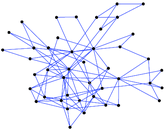### Degree distribution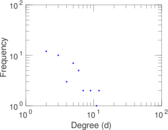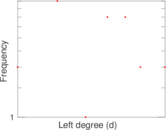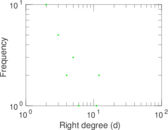### Cumulative degree distribution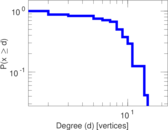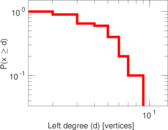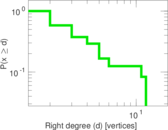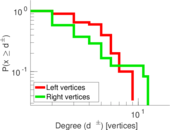### Lorenz curve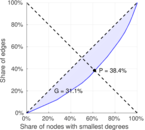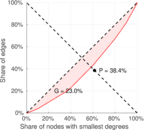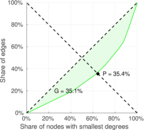### Spectral distribution of the adjacency matrix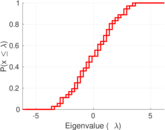### Spectral distribution of the normalized adjacency matrix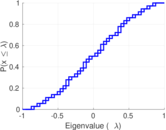### Spectral distribution of the Laplacian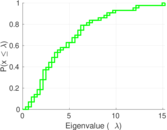### Spectral graph drawing based on the adjacency matrix### Spectral graph drawing based on the Laplacian### Spectral graph drawing based on the normalized adjacency matrix### Degree assortativity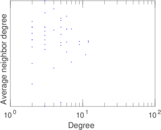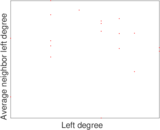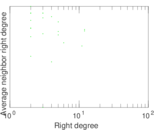### Zipf plot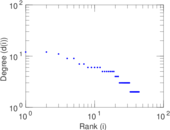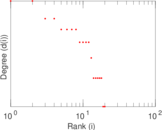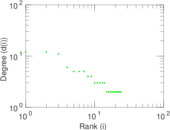### Hop distribution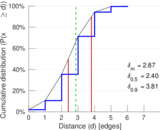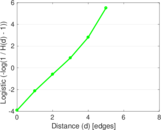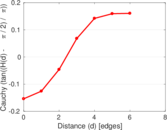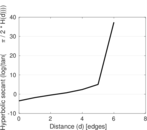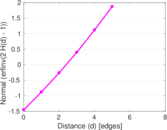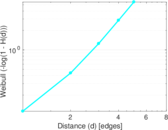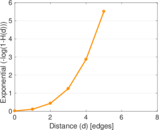### Double Laplacian graph drawing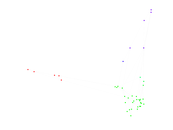### Delaunay graph drawing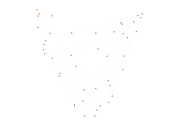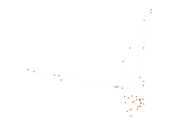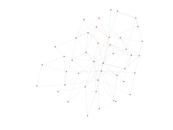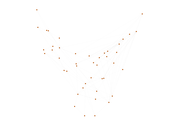### Matrix decompositions plots